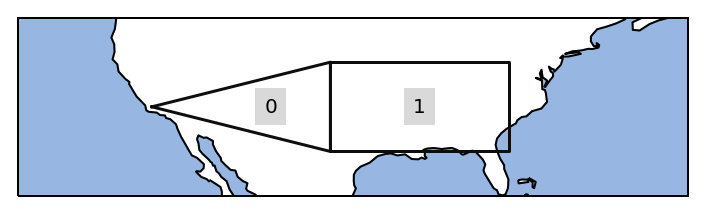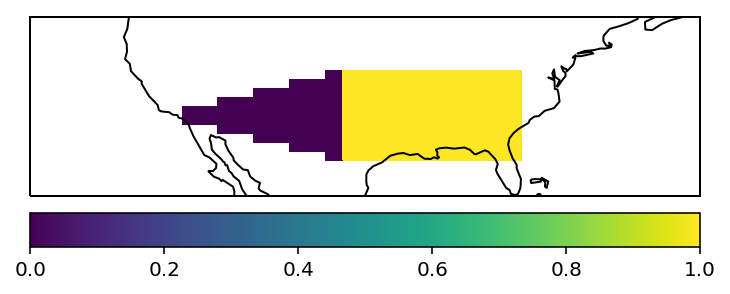Note

This tutorial was generated from an IPython notebook that can be downloaded here.

Creating own regions is straightforward.

Import regionmask and check the version:

import regionmask

'0.4.0'


Assume you have two custom regions in the US.

US1 = [[-100., 30], [-100, 40], [-120, 35]]
US2 = [[-100., 30], [-80, 30], [-80, 40], [-100, 40]]


You also need to provide numbers, names and abbreviations:

numbers = [0, 1]
names = ['US_west', 'US_east']
abbrevs = ['USw', 'USe']



Again we can plot the outline of the defined regions

ax = USmask.plot() #(label='abbrev')

import cartopy.crs as ccrs
# fine tune the extent
ax.set_extent([225, 300, 25, 45], crs=ccrs.PlateCarree());import numpy as np

# define lat/ lon grid
lon = np.arange(200.5, 330)
lat = np.arange(74.5, 15, -1)

# for the plotting
lon_edges = np.arange(200, 330)
lat_edges = np.arange(74, 14, -1)


import matplotlib.pyplot as plt

ax = plt.subplot(111, projection=ccrs.PlateCarree())
# pcolormesh does not handle NaNs, requires masked array

h = ax.pcolormesh(lon_edges, lat_edges, mask_ma, transform=ccrs.PlateCarree(), cmap='viridis')

ax.coastlines()

ax.set_extent([225, 300, 25, 45], crs=ccrs.PlateCarree());## Use shapely Polygon¶

If you have the region defined as a shapely polygon, this also works:

from shapely.geometry import Polygon

US1_poly = Polygon(US1)
US2_poly = Polygon(US2)

USmask_poly = regionmask.Regions_cls('USmask', numbers, names, abbrevs, [US1_poly, US2_poly])